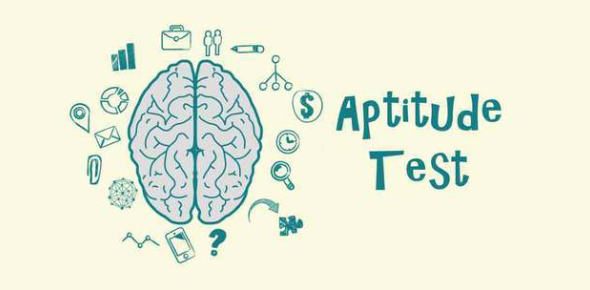# Interesting Quiz On Aptitude Test! Trivia

15 Questions | Total Attempts: 162Settings.

• 1.
Rajeev’s age after 15 years will be 5 times his age 5 years back. What is the present age of him?
• A.

16 years

• B.

10 years

• C.

15 years

• D.

12 years

• 2.
Sachin is younger than Rahul by 4 years. If their ages are in the respective ratio of 7:9, how old is Sachin?
• A.

14 years

• B.

24 years

• C.

34 years

• D.

44 years

• 3.
A train 280 m long, running  with a speed of 63km/hr will pass a tree in (sec)
• A.

12 sec

• B.

16 sec

• C.

17 sec

• D.

20 sec

• 4.
The difference between a positive fraction and  its reciprocal is 9/20.The fraction is
• A.

6/5

• B.

4/9

• C.

1/5

• D.

4/5

• 5.
The average of 20 numbers is zero of them at the most how many may be greater than zero?
• A.

10

• B.

15

• C.

19

• D.

124

• 6.
Look at this series: 21, 9, 21, 11, 21, 13, 21, ... What number should come next?
• A.

18

• B.

24

• C.

8

• D.

15

• 7.
If log10 2 = 0.3010, then log2 10 is equal to:
• A.

1000/30

• B.

1000/301

• C.

10/301

• D.

1000/3

• 8.
QAR, RAS, SAT, TAU, _____
• A.

UAV

• B.

RAV

• C.

UTS

• D.

TAR

• 9.
Class A has a higher enrollment than Class B. Class C has a lower enrollment than Class B. Class A has a lower enrollment than Class C. If the first two statements are true, the third statement is
• A.

True

• B.

False

• C.

Not sufficient data

• D.

None

• 10.
Pointing to a man in the photograph, a woman said,” His brother’s father is the only son of my grandfather.’ How is the woman related to the man in the photograph?
• A.

Sister

• B.

Mother

• C.

Aunt

• D.

Grand Mother

• 11.
If FRIEND is coded as HUMJTK, how cam CANDLE be written in that code?
• A.

HLRIRL

• B.

EDJEIM

• C.

EDRIRL

• D.

EDRILU

• 12.
If selling price is doubled, the profit triples. Find the profit percent.
• A.

50%

• B.

0%

• C.

100%

• D.

75%

• 13.
If three numbers are in the ratio 3:2:5 be such that the sum of their squares is 1862, the middle number will be?
• A.

15

• B.

14

• C.

16

• D.

17

• 14.
The odds against the occurrence of an event are 5:4.; the probability of its occurrence is:
• A.

4/5

• B.

4/9

• C.

5/9

• D.

2/5

• 15.
If ‘A’ is even, which of the following is true?
• A.

A+1 is always Even

• B.

A+4 is always odd

• C.

3A+5 is always divisible by 11

• D.

3A is always divisible by 6

Related TopicsBack to top